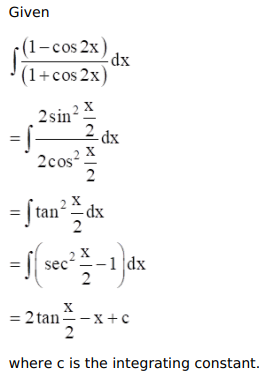# Mark against the correct answer in each of the following:

Question:

Mark $(\sqrt{ })$ against the correct answer in each of the following:

$\int \frac{(1-\cos 2 x)}{(1+\cos 2 x)} d x=?$

A. $\tan x+x+C$

B. $\tan x-x+C$

C. $-\tan x+x+C$

D. none of these

Solution: